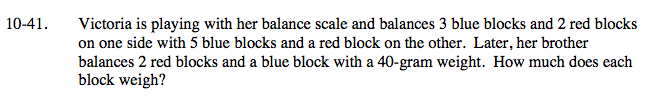### Home > A2C > Chapter 10 > Lesson 10.1.2 > Problem10-41

10-41.Create a system of equations to solve the problem.

Where r is the weight of a red block, and b is the weight of a blue block:

3b + 2r = 5b + r

2r + b = 40